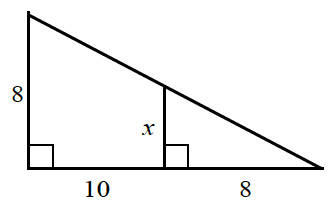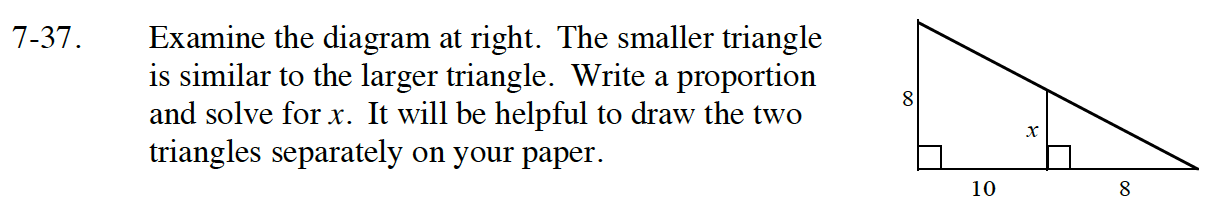Home > CC4 > Chapter 7 > Lesson 7.1.3 > Problem7-37

7-37.Examine the diagram at right. The smaller triangle is similar to the larger triangle. Write a proportion and solve for x. It will be helpful to draw the two triangles separately on your paper. Homework Help ✎Draw two separate triangles. The larger triangle has base 10 + 8 = 18.

$\frac{x}{8} = \frac{8}{18}$